# Difference between revisions of "Linear representation theory of groups of order 32"

This article gives specific information, namely, linear representation theory, about a family of groups, namely: groups of order 32.
View linear representation theory of group families | View linear representation theory of groups of a particular order |View other specific information about groups of order 32

## Degrees of irreducible representations

FACTS TO CHECK AGAINST FOR DEGREES OF IRREDUCIBLE REPRESENTATIONS OVER SPLITTING FIELD:
Divisibility facts: degree of irreducible representation divides group order | degree of irreducible representation divides index of abelian normal subgroup
Size bounds: order of inner automorphism group bounds square of degree of irreducible representation| degree of irreducible representation is bounded by index of abelian subgroup| maximum degree of irreducible representation of group is less than or equal to product of maximum degree of irreducible representation of subgroup and index of subgroup
Cumulative facts: sum of squares of degrees of irreducible representations equals order of group | number of irreducible representations equals number of conjugacy classes | number of one-dimensional representations equals order of abelianization

### Full listing

Here are the degrees of irreducible representations for all groups of order 32:

### Grouping by Hall-Senior families

FACTS TO CHECK AGAINST FOR IRREDUCIBLE REPRESENTATIONS AND ISOCLINISMS
Background: isoclinic groups
Basic fact: Isoclinic groups have same proportions of degrees of irreducible representations

Note that isoclinic groups have same proportions of degrees of irreducible representations, and in particular isoclinic groups of the same order have precisely the same degrees of irreducible representations. Thus, all groups in the same Hall-Senior family have the same degrees of irreducible representations. However, the same multiset of degrees of irreducible representations could be attained by more than one Hall-Senior family, as we see in the table below.

For the first three Hall-Senior families$\Gamma_1,\Gamma_2,\Gamma_3$, there are isoclinic groups of smaller order, hence the degrees of irreducible representations can be computed by first computing the degrees of irreducible representations of those isoclinic groups of smaller order and then scaling up the proportions based on the order. For instance, dihedral group:D8 of order 8 and family$\Gamma_2$ has 4 irreps of degree 1 and 1 of degree 2, so the groups in family$\Gamma_2$ and of order 32 have$4 \times (32/8) = 16$ irreps of degree 1 and$1 \times (32/8) = 4$ irreps of degree 2.

For more background on the Hall-Senior families business, see Groups of order 32#Families and classification.

Family name Isomorphism class of inner automorphism group Isomorphism class of derived subgroup Number of members Nilpotency class Members Second part of GAP ID of members (sorted ascending) Hall-Senior numbers of members (sorted ascending) Number of irreps of degree 1 (= order of abelianization) Number of irreps of degree 2 Number of irreps of degree 4 Total number of irreps Smallest order of group isoclinic to it Degrees of irreps of smallest order isoclinic stem group$\Gamma_1$ trivial group trivial group 7 1 all abelian groups of order 32: [SHOW MORE] [SHOW MORE] 1-7 32 0 0 32 1 1$\Gamma_2$ Klein four-group cyclic group:Z2 15 2 [SHOW MORE] [SHOW MORE] 8-22 16 4 0 20 8 1 (4 times), 2 (1 time)$\Gamma_3$ dihedral group:D8 cyclic group:Z4 10 3 [SHOW MORE] [SHOW MORE] 23-32 8 6 0 14 16 1 (4 times), 2 (3 times)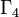$\Gamma_4$ elementary abelian group:E8 Klein four-group 9 2 [SHOW MORE] 27-35 33-41 8 6 0 14 32 1 (8 times), 2 (6 times)$\Gamma_5$ elementary abelian group:E16 cyclic group:Z2 2 2 extraspecial groups: [SHOW MORE] 49, 50 42, 43 16 0 1 17 32 1 (16 times), 4 (1 time)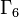$\Gamma_6$ direct product of D8 and Z2 cyclic group:Z4 2 3 [SHOW MORE] 43,44 44,45 8 2 1 11 32 1 (8 times), 2 (2 times), 4 (1 time)$\Gamma_7$ SmallGroup(16,3) Klein four-group 3 3 faithful semidirect product of E8 and Z4, SmallGroup(32,7), SmallGroup(32,8) 6-8 46-48 8 2 1 11 32 1 (8 times), 2 (2 times), 4 (1 time)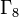$\Gamma_8$ dihedral group:D16 cyclic group:Z8 3 4 maximal class groups: [SHOW MORE] 18-20 49-51 4 7 0 11 32 1 (4 times), 2 (7 times)

### Grouping by degrees of irreducible representations

Note that since number of conjugacy classes in group of prime power order is congruent to order of group modulo prime-square minus one, all the values for the total number of irreducible representations, which equals the number of conjugacy classes, is congruent to 32 mod 3, and hence congruent to 2 mod 3.

As noted above, isoclinic groups have same proportions of degrees of irreducible representations, and in particular isoclinic groups of the same order have precisely the same degrees of irreducible representations. Thus, all groups in the same Hall-Senior family have the same degrees of irreducible representations. However, the same multiset of degrees of irreducible representations could be attained by more than one Hall-Senior family, as we see in the table below.

For more background on the Hall-Senior families business, see Groups of order 32#Families and classification.

For more on the subgroup structure stuff (the two right-most columns) see subgroup structure of groups of order 32#Abelian subgroups.

Number of irreps of degree 1 (= order of abelianization) Number of irreps of degree 2 Number of irreps of degree 4 Total number of irreps (= number of conjugacy classes) Total number of groups Nilpotency class(es) attained by these List of groups List of GAP IDs (ascending order) List of Hall-Senior numbers (ascending order) List of Hall-Senior families (equivalence classes under isoclinism) Order of inner automorphism group (bounds square of degree of irreducible representation) Minimum possible index of abelian normal subgroup (degree of irreducible representation divides index of abelian normal subgroup)
32 0 0 32 7 1 [SHOW MORE] [SHOW MORE] 1--7$\Gamma_1$ (abelian groups) 1 1
16 4 0 20 15 2 PLACEHOLDER FOR INFORMATION TO BE FILLED IN: [SHOW MORE] [SHOW MORE] 8--22$\Gamma_2$ 4 2
8 6 0 14 19 2,3 PLACEHOLDER FOR INFORMATION TO BE FILLED IN: [SHOW MORE] [SHOW MORE] 23--41$\Gamma_3$ and$\Gamma_4$ 8 2
16 0 1 17 2 2 [SHOW MORE] 49, 50 42, 43$\Gamma_5$ (extraspecial groups) 16 4
8 2 1 11 5 3 PLACEHOLDER FOR INFORMATION TO BE FILLED IN: [SHOW MORE] 6, 7, 8, 43, 44 44--48$\Gamma_6$ and$\Gamma_7$ 16 4
4 7 0 11 3 4 [SHOW MORE] 18, 19, 20 49--51$\Gamma_8$ (maximal class groups) 16 2

Here is the GAP code to generate this information:

### Correspondence between degrees of irreducible representations and conjugacy class sizes

For groups of order 32, it is true that the list of conjugacy class sizes completely determines the list of degrees of irreducible representations, and vice versa. The details are given below. The middle column, which is the total number of each, separates the description of the list of conjugacy class sizes and the list of degrees of irreducible representations:

Number of conjugacy classes of size 1 Number of conjugacy classes of size 2 Number of conjugacy classes of size 4 Number of conjugacy classes of size 8 Total number of conjugacy classes = number of irreducible representations Number of irreps of degree 1 Number of irreps of degree 2 Number of irreps of degree 4
32 0 0 0 32 32 0 0
8 12 0 0 20 16 4 0
2 15 0 0 17 16 0 1
4 6 4 0 14 8 6 0
2 3 6 0 11 8 2 1
2 7 0 2 11 4 7 0

Note that the phenomenon of the conjugacy class size statistics and degrees of irreducible representations determining one another is not true for all orders:

## Splitting field

### Rationals and reals: properties

Group GAP ID second part Hall-Senior number Hall-Senior symbol Nilpotency class rational-representation group (all representations realized over rationals)? rational group (all characters take rational values)? real-representation group (all representations realized over reals)? ambivalent group (all characters take real values)?
Cyclic group:Z32 1 7$(5)$ 1 No No No No
SmallGroup(32,2) 2 18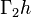$\Gamma_2h$ 2 No No No No
Direct product of Z8 and Z4 3 5$(32)$ 1 No No No No
Semidirect product of Z8 and Z4 of M-type 4 19$\Gamma_2i$ 2 No No No No
SmallGroup(32,5) 5 20$\Gamma_2j_1$ 2 No No No No
Faithful semidirect product of E8 and Z4 6 46$\Gamma_7a_1$ 3 No No No No
SmallGroup(32,7) 7 47$\Gamma_7a_2$ 3 No No No No
SmallGroup(32,8) 8 48$\Gamma_7a_3$ 3 No No No No
SmallGroup(32,9) 9 27$\Gamma_3c_1$ 3 No No No No
SmallGroup(32,10) 10 28$\Gamma_3c_2$ 3 No No No No
Wreath product of Z4 and Z2 11 31$\Gamma_3e$ 3 No No No No
Nontrivial semidirect product of Z4 and Z8 12 21$\Gamma_2j_2$ 2 No No No No
Semidirect product of Z8 and Z4 of semidihedral type 13 3 No No No No
Semidirect product of Z8 and Z4 of dihedral type 14 3 No No No No
SmallGroup(32,15) 15 32$\Gamma_3f$ 3 No No No No
Direct product of Z16 and Z2 16 6$(41)$ 1 No No No No
M32 17 22$\Gamma_2k$ 2 No No No No
Dihedral group:D32 18 49$\Gamma_8a_1$ 4 No No Yes Yes
Semidihedral group:SD32 19 50$\Gamma_8a_2$ 4 No No No No
Generalized quaternion group:Q32 20 51$\Gamma_8a_3$ 4 No No No Yes
Direct product of Z4 and Z4 and Z2 21 3$(2^21)$ 1 No No No No
Direct product of SmallGroup(16,3) and Z2 22 11$\Gamma_2c_1$ 2 No No No No
Direct product of SmallGroup(16,4) and Z2 23 12$\Gamma_2c_2$ 2 No No No No
SmallGroup(32,24) 24 16$\Gamma_2f$ 2 No No No No
Direct product of D8 and Z4 25 14$\Gamma_2e_1$ 2 No No No No
Direct product of Q8 and Z4 26 15$\Gamma_2e_2$ 2 No No No No
SmallGroup(32,27) 27 33$\Gamma_4a_1$ 2  ? Yes  ? Yes
SmallGroup(32,28) 28 36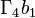$\Gamma_4b_1$ 2 No No No No
SmallGroup(32,29) 29 37$\Gamma_4b_2$ 2 No No No No
SmallGroup(32,30) 30 38$\Gamma_4c_1$ 2 No No No No
SmallGroup(32,31) 31 39$\Gamma_4c_2$ 2 No No No No
SmallGroup(32,32) 32 40$\Gamma_4c_3$ 2 No No No No
SmallGroup(32,33) 33 41$\Gamma_4d$ 2 No No No No
Generalized dihedral group for direct product of Z4 and Z4 34 34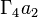$\Gamma_4a_2$ 2 Yes Yes Yes Yes
SmallGroup(32,35) 35 35$\Gamma_4a_3$ 2  ? Yes  ? Yes
Direct product of Z8 and V4 36 4$(31^2)$ 1 No No No No
Direct product of M16 and Z2 37 13$\Gamma_2d$ 2 No No No No
Central product of D8 and Z8 38 17$\Gamma_2g$ 2 No No No No
Direct product of D16 and Z2 39 23$\Gamma_3a_1$ 3 No No Yes Yes
Direct product of SD16 and Z2 40 24$\Gamma_3a_2$ 3 No No No No
Direct product of Q16 and Z2 41 25$\Gamma_3a_3$ 3 No No No Yes
Central product of D16 and Z4 42 26$\Gamma_3b$ 3 No No No No
Holomorph of Z8 43 44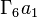$\Gamma_6a_1$ 3  ? Yes  ? Yes
SmallGroup(32,44) 44 45$\Gamma_6a_2$ 3  ? Yes  ? Yes
Direct product of E8 and Z4 45 2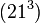$(21^3)$ 1 No No No No
Direct product of D8 and V4 46 8$\Gamma_2a_1$ 2 Yes Yes Yes Yes
Direct product of Q8 and V4 47 9$\Gamma_2a_2$ 2 No Yes No Yes
Direct product of SmallGroup(16,13) and Z2 48 10$\Gamma_2b$ 2 No No No No
Inner holomorph of D8 49 42$\Gamma_5a_1$ 2  ? Yes  ? Yes
Central product of D8 and Q8 50 43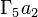$\Gamma_5a_2$ 2  ? Yes  ? Yes
Elementary abelian group:E32 51 1$(1^5)$ 1 Yes Yes Yes Yes